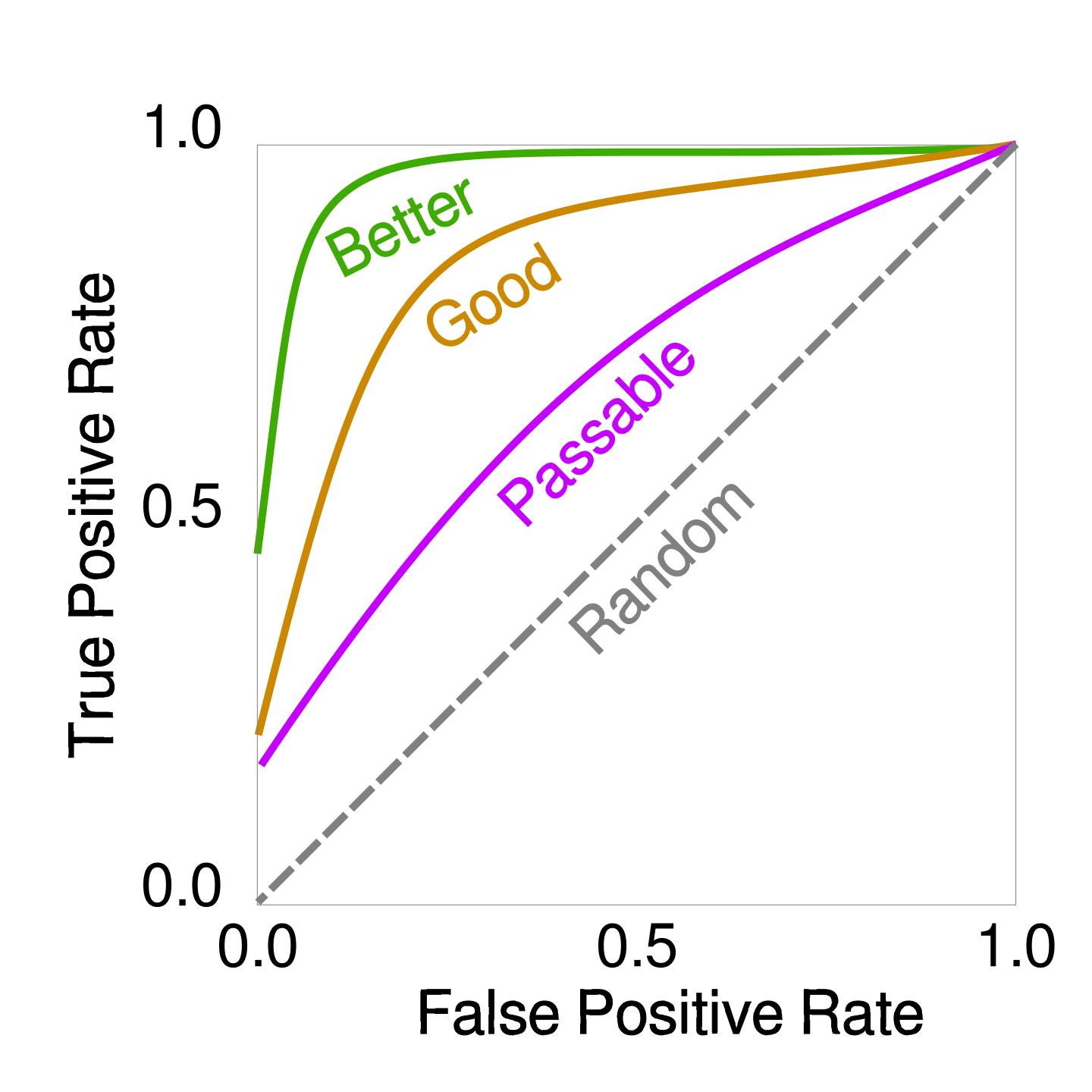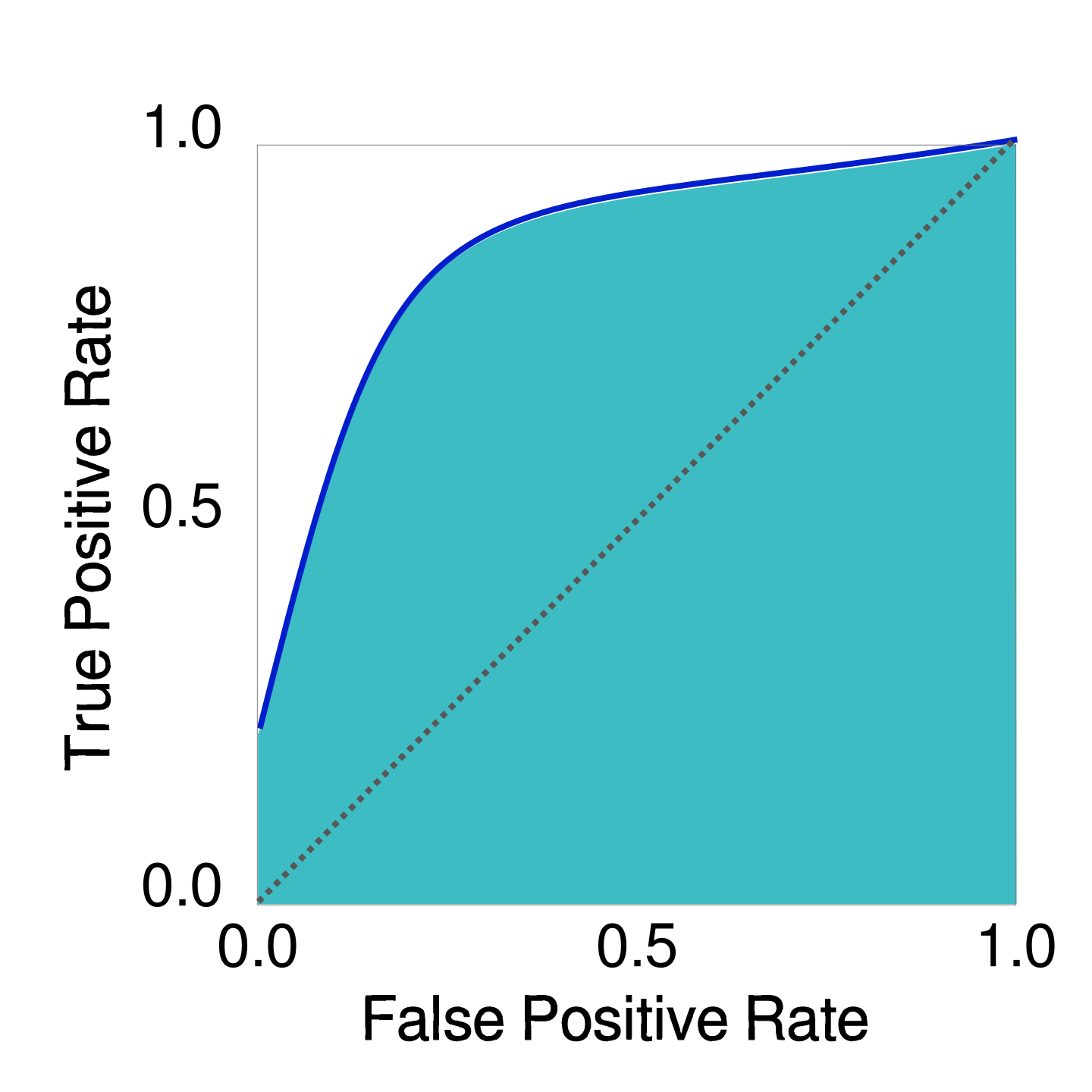# Classification Model Evaluation: Making Sense of Some Common Methods

So, you've trained your classification model. Now you have a randomized, unlabeled test data set, and you need to decide which metrics to use to make sure your model is performing well. There are an overwhelming number of choices available in sklearn.metrics. For this post, I will discuss the pros and cons of a few of the most common methods: accuracy, precision, recall, and Area Under the Curve (AUC). Each of these methods produces a scalar result -- a number between 0 and 1, with 1 being the best score.

For the sake of simplicity, I will discuss some examples of binary prediction models, but these methods can also be used for multi-class and multi-label data sets.

# Accuracy: The Simplest Metric

Of these methods, accuracy (sklearn.metrics.accuracy_score) is the easiest to understand, as it refers to a simple average. It aims to answer the question: Out of all the possible predictions, how many were correct? The calculation is simple: divide the number of correct predictions by the total number of predictions. The advantage of this method is that it’s simple to explain to your stakeholders, but is it all that useful?

Take a binary classification that provides 90 positives and 10 negatives. Say your model predicts 100 positives. You will have 90 true positives, and 10 false positives. Despite an accuracy of 90%, it is not a good model. This skewed binary classification is an example of a “class-imbalanced data set.” For these class-imbalanced data sets, there are some metrics that work better: precision and recall.

# Precision: Reducing False Positives

Precision (sklearn.metrics.precision_score), also called “positive predictive value,” measures the proportion of positive identifications that are actually correct. Consider a prediction model that classifies emails as “spam” or “not spam.” Precision measures how many of the “spam” predictions were correctly categorized as “spam.” Since it can be annoying or even disastrous if an important email is mislabeled and sent to the abyss of the spam folder, you want to reduce false positives for this model. This is when you want to take a look at your precision metric. To manually calculate precision, you would divide true positives (tp) by the sum of true positives (tp) and false positives (fp). The resulting score will be between 0 and 1, with 1 being the best score.# Recall: Reducing False Negatives

On the other hand, maybe you would rather predict some false positives, as long as the model correctly captures every positive result. Medical screening tests are a good example of such a scenario. You would rather have a false positive, in which case the patient goes for further testing (even if the final result ends up being negative), than to miss a positive diagnosis. In this case, you’d want to measure recall, which is also called sensitivity or True Positive Rate. Recall (sklearn.metrics.recall_score) takes the ratio of correctly predicted positive observations (true positives) to all of the results that were actually positive. To manually calculate recall, take the ratio of true positives (tp) vs. the sum of true positives (tp) and false negatives (fn). The result is a number between 0 and 1, with 1 being the best score.# Accounting for Classification Thresholds

Suppose you want to take a more balanced approach and minimize the chances of both false positives and false negatives. One such example might be a fraud detection model. You don’t want any false positives that would prevent an account holder from conducting business. You also want to minimize false negatives that would allow fraudulent charges.

You can adjust precision and recall scores by manipulating the classification threshold. If you raise your classification threshold, in general you will reduce false positives, increasing precision. At the same time, you will cause the number of true positives to decrease or stay the same and the number of false negatives will increase or stay the same. Thus, recall will either stay constant or decrease.

Calibrating both precision and recall across all of the possible classification thresholds would be quite a burden. Fortunately, sklearn offers some methods that do this work for you: ROC Curve and AUC.

## ROC Curve

The Receive Operator Characteristic (ROC) curve (sklearn.metrics.roc_curve) plots the true positive rate (tpr) against the false positive rate (fpr) across all classification thresholds. If your model is performing well, the curve will be located towards the top-left corner of the plot, indicating a high true positive rate and low false positive rate across different threshold values.

Ideally, your model would consistently give a high true positive rate across all thresholds, and thus its ROC curve would look like the one labeled “better” in the diagram below:

#### Sample ROC CurvesThe dotted line shows the ROC of a perfectly random classification. Obviously, we want to be better than this: An ROC lower than the dotted line would mean that our classifier is worse than a random cast of the die.

Intuitively, we see that the faster the ROC curve climbs, the higher the model quality is. Now, let’s formalize this intuition. The “climb fast and stay there” feature of the curve can be measured as the area under the curve, or AUC.

## Area Under the Curve (AUC)

The AUC (sklearn.metrics.roc_auc_score) is a value between 0 and 1 that scores the ability of a model to distinguish between positive and negative classes across all possible thresholds. You can use this number to compare the performance of different versions of your model, with higher values indicating better performance.

#### Sample AUCNote the dotted line for random classification that bisects the plot. Since the area of the whole diagram is 1, the area under the random classification line is 0.5.  A score between 0 and 0.5 indicates that the model performs worse than random chance, which is obviously bad. A score between 0.5 and 1 measures the degree to which the model performs better than random chance.

To sum it up, accuracy offers a simplified picture of the model's correctness, precision focuses on the accuracy of positive predictions, recall measures the ability to capture all positive instances, and AUC assesses a model’s overall ability to discriminate between classes. The choice of metric depends on the specific problem, whether it is a class-imbalanced data set, and the relative importance of minimizing false positives and/or false negatives.